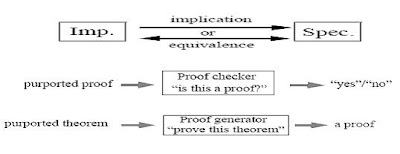13 October 2007

Theorem proving

A theorem in logic is nothing but relationship (i.e. logical equivalence/logical implication) between a specification and an implementation. This theorem has to be proven within the context of a proof calculus. The implementation should satisfy specifications provided. This is then proved by mathematical reasoning. Implementation and specification expressed as formulas in a formal logic. The implementation provides axioms and assumptions that the proof can draw upon. The inference can be simplification, rewriting, induction etc.Figure (1) Theorem proving 

Proof checking and generation
It is a purely syntactic matter to decide whether each theorem is an axiom or follows from previous theorems (axioms) by a rule of inference. Complete automation of theorem proving methodology is generally not possible due to theoretical limitations of decisions. However, a greater part of the theorem, like decidable subsets, specific classes of applications and specification styles can be automated. All theorem proving techniques require human guidance in indirect form.

Theorem Proving Systems
Theorem proving systems can be broadly classified into Automated Deduction Systems (e.g. Prolog) and Interactive Theorem Proving Systems. Automated deduction systems are full automatic, but only for a decidable subset of FOL. here speed is emphasized over versatility. Since this method is often developed in the context of application specific research, methodology is implemented by ad hoc decision procedures. Interactive theorem proving systems are semi-automatic, but not restricted to a decidable subset. Versatility of the method is emphasized over speed. Naturally this method is slow. Theoretically a complete proof can be generated for every theorem.

Some known theorem proving systems are Boyer-Moore (first-order logic), HOL (higher-order logic), PVS (higher-order logic) and Lambda (higher-order logic).

Advantages of Theorem Proving
* Theorem proving methodology provides high abstraction and powerful logic expressiveness and powerful reasoning.
* User is in full control of the design. Hence hierarchical structure and regularity of the design ca be fully exploited.
* The methodology is very much useful for verifying parameterized Datapath-dominated circuits. The technique can be customized for specific application i.e. larger proofs can be built based on basic ones.

Disadvantages of Theorem Proving

* User intervention and guidance is necessary for the verification process. This necessitates expertise in the field. To prove small theorems large human investment is necessary.
* The methodology is automated for narrow classes of designs only. Hence the technique is still under development.
* As the complexity of the design increases theorem proving becomes difficult.

Reference

 Sandeep K. Shukla, “An Introduction to Formal Verification”, http://www.ics.uci.edu/~rgupta/ics212/w2002/verification.pdf, 9/10/07

 Rolf Drechsler, “Towards Formal Verification on the System Level”, Institute of Computer Science, University of Bremen, Germany, 2005

 Prof. Eduard Cerny, Xiaoyu Song, “Formal Verification Methods”, University of Montrial, Canada, 1999. http://www.iro.umontreal.ca/~cerny/IFT6222/1-intro-99.0808.pdf, 9/10/07

 Jawahar Jain, Amit Narayan, M.Fujita, A.Sangiovanni Vincentelli, “A Survey of Techniques for Formal Verification of Combinational Circuits”, Proceedings of the 1997 International Conference on Computer Design (ICCD '97), IEEE, 1997

 F. Wang, “Formal Verification of Timed Systems: A Survey and Perspective”, Proceedings of the IEEE, Vol. 92, No. 8, August 2004. pp.283-1305. http://www.cerc.utexas.edu/~jay/fv_surveys/, 9/10/07

 J. Bhadra, M. S. Abadir, L.-C. Wang, S. Ray, “Functional Verification through Hybrid Techniques: A Survey”, journal of IEEE Design & Test of Computers, March-April 2007. http://www.cerc.utexas.edu/~jay/fv_surveys/, 9/10/07

 M. Prasad, A. Biere, A. Gupta, “A Survey of Recent Advances in SAT-Based Formal Verification”, Software Tools for Technology Transfer, Vol. 7, No. 2, 2005. pp. 156-173. http://www.cerc.utexas.edu/~jay/fv_surveys/, 9/10/07

Related Articles